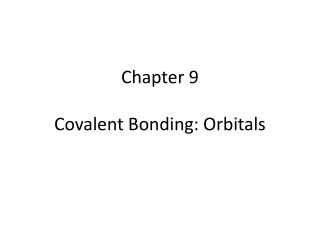# Chapter 9 Covalent Bonding: Orbitals - PowerPoint PPT PresentationDownload PresentationChapter 9 Covalent Bonding: Orbitals

Chapter 9 Covalent Bonding: OrbitalsDownload Presentation## Chapter 9 Covalent Bonding: Orbitals

- - - - - - - - - - - - - - - - - - - - - - - - - - - E N D - - - - - - - - - - - - - - - - - - - - - - - - - - -
##### Presentation Transcript

1. Chapter 9Covalent Bonding: Orbitals

2. 9.1 – Hybridization and the localized electron model • Localized electron model: • Widely used • Easy* *easy is a relative term – 9.1 will not be an “easy” section

3. 9.1 – Hybridization and the localized electron model • Valence orbitals are the orbitals associated with the highest principal quantum level that contains electrons in an atom. For example, in methane: For the hydrogen atom, the 1s orbital is the valence (bonding) orbital For the carbon atom, the 2s and 2p orbitals are the valence (bonding) orbitals

4. 9.1 – Hybridization and the localized electron model • If we accepted this, we would also have to accept: • The C-H bonds in methane would be different, since some are formed from 1s-2s orbitals, while others are formed from 1s-2p orbitals. • There may only be two C-H bonds formed because there are only two electrons in the 2p orbital of carbon that are free for bonding (the 2s are happy!) • Since the 2p lobes are perpendicular to one another, we would expect the 3 lobes to be at 90o to one another (we know they’re really 109.5o).

5. 9.1 – Hybridization and the localized electron model • None of these actually happen! • … So what does? • Hybridization!

6. 9.1 – Hybridization and the localized electron model • sp3 hybridization • When an atom creates a hybrid orbital • called sp3 orbitals because they are formed from 1s and 3p orbitals (s1p3)

7. 9.1 – Hybridization and the localized electron model • sp3 hybridization

8. 9.1 – Hybridization and the localized electron model • sp3 hybridization • Whenever a set of equivalent tetrahedral atomic orbitals is required by an atom, this model assumes that the atom adopts a set of sp3 orbitals (109.5o). • Examples, the C in CH4, the O in H2O • The atom becomes sp3 hybridized

9. 9.1 – Hybridization and the localized electron model • sp2 hybridization • Recall that double bonds act as one electron “group” • In ethene, each carbon molecule has 3 “groups” • The geometry of each carbon is trigonal planar (120o), so sp3 hybrids will not fit here (they require 109.5o). • sp2 hybrids form, instead, with angles of 120o

10. 9.1 – Hybridization and the localized electron model • sp2 hybridization

11. 9.1 – Hybridization and the localized electron model • sp2 hybridization • Whenever an atom is surrounded by three electron groups, it adopts a sp2 hybridization

12. 9.1 – Hybridization and the localized electron model • sigma vs pi bonds: • A double bond is always composed of a sigma (σ) bond and a pi (π)bond. • A sigma bond is created by the overlap of the hybridized orbitals in line with the two atoms • A pi bond is created by the overlap of the unhybridized p orbitals, above and below the sigma bond.

13. 9.1 – Hybridization and the localized electron model • sigma vs pi bonds:

14. 9.1 – Hybridization and the localized electron model • sp hybridization • Following this pattern, if an atom has two electron groups around it, it adopts a sp hybridization:

15. 9.1 – Hybridization and the localized electron model • sp hybridization • Example, CO2 (or HCN, HCCH, etc…) 2 pi bonds and 2 sigma bonds

16. 9.1 – Hybridization and the localized electron model • dsp3 hybridization • Applies to atoms that exceed the octet rule and have 5 electron groups • A hybridization of 1d orbital, 1s orbital, and 3p orbitals (in a trigonalbipyramidal arrangement) • Example, PCl5

17. 9.1 – Hybridization and the localized electron model • d2sp3 hybridization • When atoms have 6 electron groups • Arrange in octahedral shape • Example SF6

18. Summary

19. 9.1 – Hybridization and the localized electron model • For each molecule (or ion) predict the hybridization of each atom and describe its structure: • A. CO • B. BF4- • C. XeF2

20. 9.2 (9.5) – Incorporating resonance • Example, benzene (C6H6) • It has alternating double bonds. That means that there is a single (sigma) bond between each carbon atom. The bond which alternates is the second (pi) bond.

21. 9.2 – The molecular orbital model • Drawbacks of localized electron model: • Assumes electrons are localized, so it does not address the concept of resonance • Does not effectively deal with molecules with unpaired electrons • Gives no direct information on bond energies

22. Quiz #9 The skeleton structure of AZT is incomplete. Re-draw the structure, completing the lone pairs to give each atom a formal charge of 0. How many sigma and pi bonds are there? Put a square around atoms that are sp3 hybridized. Circle the atom that is sp hybridized